Previous: Fixed Static Pressure Family Up: Fixed Static Pressure Family Next: Zero Gradient Family of
This is an automatically generated documentation by LaTeX2HTML utility. In case of any issue, please, contact us at info@cfdsupport.com.

## fixedValue Static Pressure Boundary Condition

fixedValue boundary condition simply prescribe fixed value for the quantity. Fixed value can be uniform or nonuniform.

p:

 boundaryField
{
".*_outlet"
{
type            fixedValue;
value           uniform 0;
}
}


## Postprocessing of the Main Simulation Results

All the important integral values are evaluated already during the simulation.

All the rotating machines has typically following integral values:

• flow rate at inlet
• flow rate at outlet
• efficiency
• torques
• pressure differences

All these integral values are typically followed using gnuplot scripts, for example:

# gnuplot plotFlowRate.gp
# gnuplot efficiency.gp
# gnuplot torques.gp

Previous: Model setting without an Up: Water turbines – notes Next: Interface between rotor and
This is an automatically generated documentation by LaTeX2HTML utility. In case of any issue, please, contact us at info@cfdsupport.com.

## Conclusion

We have seen there are two basic approaches to water turbine calculations.
1. With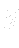in the momentum equation
1. physical setting
2. model setting
2. Without explicitin the momentum equation
Following table shows possible boundary conditions for the pressure variable
Table: Boundary conditions
 pressure setting inlet outlet, physical hTP =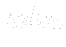fMV =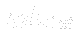, model hTP =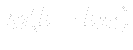fMV =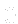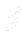hTP =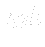fMV =We note that hTP stands for hydrostaticTotalPressure boundary condition and values listed in the Tablefor this type represent values of hydrostatic pressure in the centre of mass of the inlet surface. Whereas fMV stands for fixedMeanValue boundary condition and values listed in the Tablefor this type represent values of static pressure in the centre of mass of the outlet surface. We add that pressureInletVelocity boundary condition is prescribed for the inlet velocity field for all of the above settings, where the velocity magnitude is computed from the difference between total and static pressure and its direction is taken as a local normal to the inlet surface (usually planar). Also zeroGradient boundary condition is prescribed for the outlet surface for all of the above settings.